# AP 10th Class Maths Bits Chapter 1 Real Numbers with Answers

Practice the AP 10th Class Maths Bits with Answers Chapter 1 Real Numbers on a regular basis so that you can attempt exams with utmost confidence.

## AP SSC 10th Class Maths Bits 1st Lesson Real Numbers with Answers

Question 1.
Find the rational number in between $$\frac { 1 }{ 2 }$$ and √1
$$\frac { 3 }{ 4 }$$

Question 2.
Write the name set of rational and ir-rational numbers.
Real numbers.Question 3.
Write the logarithmic form of 35 = 243.
log3243 = 5

Question 4.
Write the symbol of “implies”.

Question 5.
Write the prime factorisation of 729.
36

Question 6.
If ‘x’ and ‘y’ are two prime numbers, then find their HCF.
1
Explanation:
HCF of any two prime numbers is always 1.

Question 7.
Find the value of log10 0.01.
-2
Explanation:
log100.01 = log10 $$\frac{1}{10^{2}}$$
= log1010-2 = – 2

Question 8.
Find the number of odd numbers in between ‘0’ and 100.
50

Question 9.
Write the exponential form of log48 = x.
4x = 8.
Explanation:
Exponential form of log48 = x is 4x = 8

Question 10.
How much the value of $$\frac{36}{2^{3} \times 5^{3}}$$ in decimal form ?
0.036.Question 11.
LCM of two numbers is 108 and their HCF is 9 and one of them is 54, then find the second one.
18
Explanation:
LCM x HCF = one number x second number
108 x 9 = 54 x second one , 108 x 9
⇒ Second one = $$\frac{108 \times 9}{54}$$ = 18.

Question 12.
$$\frac { 3 }{ 8 }$$ is example for decimal.
Terminating decimal.

Question 13.
If $$\mathbf{a} \sqrt{\mathbf{c}}=\sqrt{\mathbf{a c}}$$ , then find the value of ‘a’, (a, c are positive integers),
a = 1

Question 14.
Find the value of 9 – $$0 . \overline{9}$$.
8
Explanation:
9 – $$\frac{9}{9}$$ = 9 – 1 = 8.

Question 15.
Write rational number that equals to $$2 . \overline{6}$$
$$\frac { 8 }{ 3 }$$

Question 16.
Write the value of log25 5.
$$\frac { 1 }{ 2 }$$
Explanation:
log255 = log551 = $$\frac{1}{2}$$log55 = $$\frac{1}{2}$$

Question 17.
The fundamental theorem of arithmetic is applicable to, which least number ?
2Question 18.
Find the last digit of 650.
6

Question 19.
Which of the following is a terminat¬ing decimal ?
A) $$\frac { 10 }{ 81 }$$
B) $$\frac { 41 }{ 75 }$$
C) $$\frac { 8 }{ 125 }$$
D) $$\frac { 3 }{ 14 }$$
C)

Question 20.
Find the value of log2 32.
5

Question 21.
Which of the following is not irrational ?
A) √2
B) √3
C) √4
D) √5
(C)

Question 22.
Find the value of log10 0.001.
-3

Question 23.
Find the number of prime factors of 36.
2 and 3
Explanation:
36 = 22 x 32
∴ Two prime numbers i.e., 2 and 3.

Question 24.
Write the exponential form of
Iog10 = -3.
10-3 = 0.001

Question 25.
Define an irrational number.
Which cannot be written in the form
of p/q where p, q ∈ Z, q ≠ 0.

Question 26.
Find the LCM of 24 and 36.
72Question 27.
Find the logarithmic form of ab = c.
logac = b.

Question 28.
If 3 log (x + 3) = log 27, then find the value of x.
0
Explanation:
3 log (x + 3) = log 27
⇒ log (x + 3)3 = log 33
⇒ x + 3 = 3 ⇒ x = 0
log3729 = x ⇒ 3x = 729 = 36 ⇒ x = 6

Question 29.
If P1 and P2 are two odd prime num-bers, such that P1 > P2, then the value of $$\mathbf{P}_{1}^{2}-\mathbf{P}_{\mathbf{2}}^{2}$$ results number
An even number.

Question 30.
Find the value of $$\log _{10} 2+\log _{10} 5$$
1

Question 31.
If log3 729 = x, then find the value of x.
6
Explanation:
log3 729 = x ⇒ 3x = 729 = 36 ⇒ x = 6

Question 32.
Write the number of digits in the fractional part of the decimal form of $$\frac{7}{40}$$.
3
Explanation:
$$\frac{7}{40}=\frac{7}{2^{3} \times 5^{1}}$$
In Denominator 2n x 5m is equal to 3.

Question 33.
Write the prime factorization of 144.
24 x 32

Question 34.
Find the number of prime factors of 72.
2

Question 35.
log3 x2 = 2, then find the value of x.
3
Explanation:
log3x2 = 2 ⇒ 32 = x2 ⇒ x = 3

Question 36.
Find the value of $$9 \sqrt{2} \times \sqrt{2}$$
18

Question 37.
Find the value of log0.1 0.01.
2
Explanation:
log 0.1 0.01 = log10-1 10-2
= $$\frac{-2}{-1}$$ log10 10 = 2

Question 38.
0.3030030003 ………………. is an ………………. number.
Irrational.Question 39.
$$\frac{27}{82}$$ is a …………. decimal.
Non-terminating

Question 40.
If log 2 = 0.30103, then find log 32.
1.50515
Explanation:
log 32 = log 25 = 5 log 2
= 5 x 0.30103 .
= 1.50515

Question 41.
Expand log 15.
log5 + log3

Question 42.
Find the value of log10 10.
1

Question 43.
Calculate the value of log8 128.
$$\frac{7}{3}$$

Question 44.
Find the value of $$5 \sqrt{5}+6 \sqrt{5}-2 \sqrt{5}[/latex[ Answer: 9√5 Question 45. 743.2111111 … is a number. Answer: Rational Question 46. Find the value of log5 125. Answer: 3 Question 47. Expand log10 [latex]\frac{125}{16}$$
3 log 5 – 4 log 2
Explanation:
log10 $$\frac{125}{16}$$ = log10 125 – log1016
= log1053 – log1024
= 3 log 5 – 4 log 2

Question 48.
Find the L.C.M of the numbers 27 x 34 x 7 and 23 x 34 x 11.
27 x 34 x 7 x 11

Question 49.
If loga ax2 – 5x + 8 = 2, then find x.
2 or 3.
Explanation:
$$\log _{a} a^{x^{2}-5 x+8}=\log _{a} a^{2}$$
{2 was write down as 2-loga a}
x2 – 5x + 8 = 2
x2 – 5x + 6 = 0
by solving equation x = 2 or 3

Question 50.
Find the value of loga $$\frac{1}{a}$$.
– 1

Question 51.
Find the value of log1 1.
Not defined.

Question 52.
If log10 0.00001 = x, then find x.
-5Question 53.
Find the value of logb a • loga b.
1.

Question 54.
16 x 64 = 4k, then find the value of k.
5
Explanation:
16 x 64 = 4k
⇒ 42 x 43 = 4k
⇒ 45 = 4k
⇒ k = 5

I’m

Question 55.
Write exponential form of log464 = 3.
43 = 64

Question 56.
Calculate the value of $$\log _{9} \sqrt{3 \sqrt{3 \sqrt{3}}}$$
$$\frac{7}{16}$$

Question 57.
If ‘m’ and ‘n’ are co-primes, then find H.C.F of m2 and n2.
1

Question 58.
$$\sqrt{5}+\sqrt{7}$$ is number.
An irrational.

Question 59.
Find the H.C.F. of the numbers
37 x 53 x 24 and 32 x 74 x 28.
24 x 32

Question 60.
$$\frac{13}{125}$$ is a ……………… decimaL
Terminating

Question 61.
Write the decimal expansion of 0.225 in its rational form.
$$\frac{9}{40}$$

Question 62.
How many prime factors are there in the prime factorization of 240.
3

Question 63.
14.381 may certain the denominator when expressed in p/q form.
23 x 53

Question 64.
By which numbers 7 x 11 x 17 +34 is divisible, write them.
17 and 79
Explanation:
Given number = 7 x 11 x 17 + 34
= 17 (7 x 11 + 2)
= 17 x 79
Given number has 17 and 79 are factors.

Question 65.
Write log$$\frac{x^{2} y^{3} z^{4}}{w^{5}}$$ in the expanded form.
2 log x + 3 log y + 4 log z – 5 log w
Explanation:
log x2y3z4 – log w5 = log x2 + log y3 + log z4 – log w5
= 2log x + 3log y + 4log z – 51og w

Question 66.
Write the logarithmic form of 122 = 144.
log12 144 = 2

Question 67.
Expand log 81 x 25.
4log 3 + 2 log 5

Question 68.
What is the L.C.M. of greatest two digit number and the greatest three digit number.
9 x 11 x 111

Question 69.
Write logarithmic form of 192 = 361.
log19361 = 2

Question 70.
3 X 5 x 7 x 11 + 35 is number.
Composite

Question 71.
Write the decimal expansion of $$\frac{101}{99}$$.
$$1 . \overline{02}$$

Question 72.
If P1, p2, p3, …………… pn are co-primes, then
their LCM is
P1p2 …………… pn

Question 73.
In the above problem find HCF.
1

Question 74.
n2 – 1 is divisible by 8, if ‘n’ is number.
An odd number.Question 75.
If x and y are any two co-primes, then find their L.C.M.
x.y

Question 76.
Write $$\frac{70}{71}$$ is which type of decimal ?
Non-terminating, repeating.

Question 77.
0.12 112 1112 11112………………is…………… type of number.
Irrational

Question 78.
Write $$\frac{123}{125}$$ is which type of decimal ?
Terminating.

Question 79.
Write the product of L.C.M. and H.C.F. of the least prime and least composite number.
8

Question 80.
$$\sqrt{2}-2$$ is…………………number.
An irrational.

Question 81.
Find the number of prime factors of 1024.
Only one number, i.e., ‘2’. (i.e., 210)

Question 82.
Write the LCM of 208 and 209.
208 x 209 (Product of even and odd number is its product)

Question 83.
Write the expansion of $$\frac{87}{625}$$ terminates after how many places ?
4 places.

Question 84.
The decimal expansion of $$\frac{87}{625}$$ terminates after how many places ?
4 places.

Question 85.
What is the H.C.F. of ‘n’ and ‘n + 1’, where ‘n’ is a natural number ?
1

Question 86.
What is the prime factorisation of 20677.
23 x 29 x 31

Question 87.
Find the HCF of 1001 and 1002.
1

Question 88.
p, q are co-primes and q = 2n . 5m, where m > n, then write the decimal expansion of p/q terminates after how many places ?
‘m’ places.

Question 89.
Write the decimal fprm of $$\frac{80}{81}$$ and write repeats after how many places ?
81 = 34, so not possible.

Question 90.
If a rational number p/q has a termi¬nating decimal, then write the prime factorisation of ‘q’ is of the form.
q = 2m . 5n

Question 91.
Write the decimal expansion of $$\frac{7}{16}$$ without actual division.
0.4375

Question 92.
In the expansion of $$\frac{123}{125}$$ terminates after how many places ?
3 places.

Question 93.
What is the L.C.M of least prime and the least composite number ?
Least composite

Question 94.
Write the decimal expansion of $$\frac{27}{14}$$
$$1.9 \overline{285714}$$Question 95.
Which type of number was $$5.6789 \overline{1}$$ ?
Rational number

Question 96.
After how many places the decimal expansion of $$\frac{23}{125}$$ terminates ?
3 places.

Question 97.
Write the type of decimal expansion of $$\frac{9}{17}$$
Non-terminating & repeating.

Question 98.
Write the period of the decimal expansion of $$\frac{19}{21}$$
904761

Question 99.
After how many digits will the deci-mal expansion of $$\frac{11}{32}$$ terminates ?
Write it.
5 places.

Question 100.
If $$\sqrt{2}$$ = 1.414, then find $$3 \sqrt{2}$$.
4.242

Question 101.
Find the value of $$\frac{3}{8}$$
0.375

Question 102.
Find the value of log 64 – log 4.
16

Question 103.
Find the value of 128 ÷ 32.
4

Question 104.
Find the value of 104.
10000

Question 105.
Find the value of $$\sqrt{\mathbf{5}}$$ .
2.236

Question 106.
Find the value of log27 9.
$$\frac{2}{3}$$

Question 107.
Find the value of | – 203 |.
203

Question 108.
Complete the rule a(b + c).
ab + ac

Question 109.
Find the value of log3 $$\frac{1}{9}$$.
-2

Question 110.
How much the LCM of 12, 15 and 21.
420

Question 111.
Find the value of loga 1, a > 0.
0

Question 112.
a + (-a) = 0 = (- a) + a is called ……………… property.
InverseQuestion 113.
Find the value of $$\sqrt{\mathbf{a}} \times \sqrt{\mathbf{b}}$$
$$\sqrt{a b}$$

Question 114.
Find the value of 55.
3125

Question 115.
Find the value of $$\frac{13}{4}$$ .
3.25

Question 116.
Find the value of $$\sqrt{12544}$$
112

Question 117.
Find the value of log61.
0

Question 118.
Find the value of log1010000.
4

Question 119.
How much the HCF of 12 and 18.
6

Question 120.
Which number has no multiplicative inverse ?
0

Question 121.
How much the LCM of 306 and 657.
22338

Question 122.
Find the value of logx $$\frac{\mathbf{a}}{\mathbf{b}}$$.
logxa – logxb

Question 123.
Find the value of log32 $$\frac{1}{4}$$

Question 124.
Find the value of $$\sqrt{2025}$$
$$\frac{-2}{5}$$

Question 125.
Find the value of $$2 \sqrt{3}+7 \sqrt{3}+\sqrt{3}$$
$$10 \sqrt{3}$$

Question 126.
Find the value of 22 x 5 x 7.
140Question 127.
Find the value of log10 100.
2

Question 128.
6n cannot end with this number. What is that number ? (When ‘n’ is a posi¬tive number).
0

Question 129.
If 2x = y and log2 y = 3 then find (x – y)2.
25

Question 130.
Find the value of log3 $$\frac{1}{27}$$.
-3

Question 131.
Expanded form of log101000.
3 log 2 + 3 log 5

Question 132.
Find the value of log2512.
9

Question 133.
Write $$\frac{3}{2}$$ (log x) – (log y) as single form.
$$\log \sqrt{\frac{x^{3}}{y^{2}}}$$

Question 134.
Find HCF of 1 and 143.
1

Question 135.
Which of the following is a correct one ?
A) N⊂Z⊂W
B) N⊂W⊂Z
C) R⊂N⊂W
D) All the above
(B)

Question 136.
Find the value of log216.
4

Question 137.
Find the value of $$\log _{7} \sqrt{49}$$
1

Question 138.
Find the value of log21024.
10

Question 139.
Find the value of log18324.
2

Question 140.
Express logarithmic form of ax = b.
logab = x .Question 141.
Find the value of $$(\sqrt{7}+\sqrt{5}) \cdot(\sqrt{7}-\sqrt{5})$$
2

Choose the correct answer satisfying the following statements.

Question 142.
Statement (A) : 6n ends with the digit zero, where ‘n’ is natural number. Statement (B): Any number ends with digit zero, if its prime factor is of the form 2m x 5n, where m, n are natural numbers.
i) Both A and B are true.
ii) A is true, B is false.
iii) A is false, B is true.
iv) Both A and B are false.
(iii)
Explanation:
6n = (2 x 3)n = 2n x 3n
Its prime factors do not contain 5n i.e., of the form 2m x 5n, where m, n are natural numbers. Here (A) is incorrect but (B) is correct.
Hence, (iii) is the correct option.

Question 143.
Statement (A) : $$\sqrt{a}$$ is an irrational number, where ‘a’ is a prime number.
Statement (B) : Square root of any prime number is an irrational number.
i) Both A and B are true.
ii) A is true, B is false.
iii) A is false, B is true.
iv) Both A and B are false.
(i)
Explanation:
As we know that square root of every prime number is an irrational number. So, both A and B are correct and B explains A. Hence (i) is the correct option.

Question 144.
Statement (A) : For any two positive integers a and b,
HCF (a, b) x LCM (a, b) – a x b.
Statement (B) : The HCF of two num-bers is 5 and their product is 150. Then their LCM is 40.
i) Both A and B are true.
ii) A is true, B is false.
iii) A is false, B is true.
iv) Both A and B are false.
(ii)
Explanation:
We have,
LCM (a, b) x HCF (a, b) = a xb LCM x 5 – 150 150
∴ LCM = $$\frac{150}{5}$$ = 30
=> LCM = 30, i.e., (B) is incorrect and (A) is correct.
Hence, (ii) is the correct option.

Question 145.
Statement (A) : When a positive inte-ger ’a’ is divided by 3, the values of re-mainder can be 0, 1 (or) 2.
Statement (B) : According to Euclid’s Division Lemma a = bq + r, where 0 ≤ r < b and ‘r’ is an integer.
i) Both A and B are true.
ii) A is true, 3 is false
iii) A is false, B is true.
iv) Both A and B are false.
(i)
Explanation:
Given positive integers A and B, there exists unique integers q and r satisfy¬ing a = bq + r where 0 ≤ r < b.
This is known as Euclid’s Division Algorithm. So, both A and B are cor¬rect and B explains A.
Hence, (i) is the correct option.

Question 146.
Statement (A): A number N when di¬vided by 15 gives the remainder 2. Then the remainder is same when N is divided by 5.
Statement (B) : $$\sqrt{3}$$ is an irrational number.
i) Both A and B are true.
ii) A is true, B is false.
iii) A is false, B is true.
iv) Both A and B are false.
(ii)Question 147.
Statement (A): $$\frac{41}{1250}$$ is a terminating decimal.
Statement (B) : The rational number p/q is a terminating decimal if q = 2m x 5n, where m, n are non-nega¬tive integers.
i) Both A and B are true.
ii) A is true, B is false.
iii) A is false, B is true.
iv) Both A and B are false.
(i)

Question 148.
Statement (A) : $$\sqrt{3}$$ is an irrational number.
Statement (B) : The square root of a prime number is an irrational.
i) Both A and B are true.
ii) A is true, B is false.
iii) A is false, B is true.
iv) Both A and B are false.
(i)
Explanation:
Clearly, both A and B are correct but B does not explain A.
Hence, (i) is correct option.

Question 149.
Statement (A) : $$\frac{27}{250}$$ is a terminating decimal.
Statement (B) : The rational number p/q is a terminating decimal, if q = (2m x 5n) for some whole number m and n.
i) Both A and B are true.
ii) A is true, B is false.
iii) A is false, B is true.
iv) Both A and B are false.
(i)

Question 150.
Statement (A): $$\frac{13}{3125}$$ is a terminating decimal fraction.
Statement (B): If q = 2n . 5m where n, m are non-negative integers, then p/q is terminating decimal fraction.
i) Both A and B are true.
ii) A is true, B is false.
iii) A is false, B is true.
iv) Both A and B are false.
(i)
Explanation:
(B) is correct.
Since the factors of the denominator 3125 is of the form 2° x 55.
∴ $$\frac{13}{3125}$$ is a terminatmg decimal.
∴ Since (A) follows from (B).
∴ Hence, (i) is the correct option.

Question 151.
Statement (A) : Denominator of 34.12345 is of the form 2m x 5n, where m, n are non-negative integers.
Statement (B) : 34.12345 is a termi-nating decimal fraction.
i) Both A and B are true.
ii) A is true, B is false.
iii) A is false, B is true.
iv) Both A and B are false.
(i)
Explanation:
(B) is clearly true.
Again 34.12345 = $$\frac{3412345}{100000}$$
= $$\frac{682469}{20000}=\frac{682469}{2^{5} \times 5^{4}}$$
Its denominator is of the form 2m x 5n
[m = 5, n = 4 are non-negative integers.]
∴ (A) is true.
Since (B) gives (A).
Hence, (i) is the correct option.

Question 152.
Statement (A): The H.C.F. of two num-bers is 16 and their product is 3072. Then their L.C.M. = 162.
Statement (B): If a, b are two positive integers, then H.C.F x L.C.M = a x b.
i) Both A and B are true.
ii) A is true, B is false.-
iii) A is false, B is true.
iv) Both A and B are false.
(iii)
Explanation:
Here (B) is true (standard result)
(A) is false.
∴ $$\frac{3072}{16}$$ = 192 ≠ 162
Hence, (iii) is the correct option.Question 153.
Statement (A) : 2 is a rational num¬ber.
Statement (B): The square roots of all positive integers are irrationals.
i) Both A and B are true.
ii) A is true, B is false.
iii) A is false, B is true.
iv) Both A and B are false.
(ii)
Explanation:
Here (B) is not true.
∴ $$\sqrt{4} \neq 2$$ which is not an irrational
number.
Clearly, (A) is true.
∴ (ii) is the correct option.
Question 154.
Statement (A) : If L.C.M. {p, q} = 30 and H.C.F. {p, q} = 5, then pq = 150.
Statement (B): L.C.M. of a, b x H.C.F. of a, b = a • b.
i) Both A and B are true.
ii) A is true, B is false.
iii) A is false, B is true.
iv) Both A and B are false.
(i)

Question 155.
Statement (A) : n2 – n is divisible by 2 for every positive integer.
Statement (B): $$\sqrt{2}$$ is a rational num¬ber.
i) Both A and B are true.
ii) A is true, B is false.
iii) A is false, B is true.
iv) Both A and B are false.
(ii)

Question 156.
Statement (A): n2 + n is divisible by 2 for every positive integer n.
Statement (B): If x and y are odd posi-tive integers, from x2 + y2 is divisible by 4.
i) Both A and B are true.
ii) A is true, B is false.
iii) A is false, B is true.
iv) Both A and B are false.
(i)

If p is prime, then $$\sqrt{\mathbf{p}}$$ is irrational and if a, b are two odd prime num-bers, then a2 – b2 is composite.

Question 157.
Is $$\sqrt{7}$$ is a rational number ?
No, it is an irrational number.

Question 158.
The results of 1192 – 1112 is a ………..
number.
Composite

L.C.M. of several fractions
= $$\frac{\text { LCM of their numerators }}{\text { HCF of their denominators }}$$
H.C.F. of several fractions = $$\frac{\text { HCF of their numerators }}{\text { LCM of their denominators }}$$Question 159.
Find the LCM of the fractions $$\frac{5}{16}, \frac{15}{24}$$ and $$\frac{25}{8}$$
$$\frac{75}{8}$$.
Explanation:
L.C.M. of $$\frac{5}{16}, \frac{15}{24}$$ and $$\frac{25}{8}$$
= $$\frac{\text { L.C.M. of numerators }}{\text { H.C.F. of denominators }}$$
L.C.M’. of 5, 15 and 25 is 75.
H.C.F. of 16, 24 and 8 is 8.
The H.C.F. of the given fractions = $$\frac{75}{8}$$

Question 160.
Find the HCF of $$\frac{2}{5}, \frac{6}{25}$$ and $$\frac{8}{35}$$.
$$\frac{2}{175}$$
Explanation:

Question 161.
Find the HCF of the fractions $$\frac{8}{21}, \frac{12}{35}$$ and $$\frac{32}{7}$$
$$\frac{4}{105}$$
[H+] ion concentration in a soap used by Sohan is 9.2 x 10-22.
Explanation:
H.C.F. of given fraction is
$$\frac{\text { H.C.F. of } 8,12,32}{\text { L.C.M. of } 21,35,7}$$
= $$\frac{4}{105}$$

Question 162.
Which mathematical concept is used to find pH of a soap ?
Logarithms.

Question 163.
How much the pH of soap used by Sohan ?
pH = 21.04.

Question 164.
Write the correct matching options :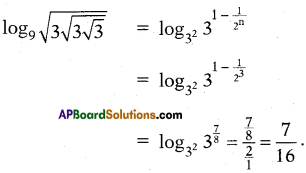A – (iii), B – (iv)

Question 165.
Write the correct matching options :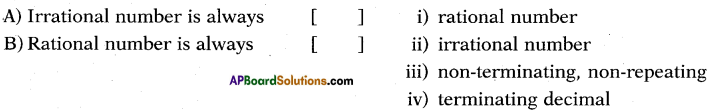A – (i), B – (ii)

Question 166.
Write the correct matching options :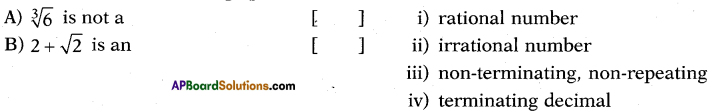A – (iii), B – (i)

Question 167.
Write the correct matching options :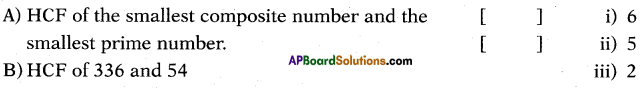A – (ii), B – (v), C – (iii)

Question 168.
Write the correct matching options: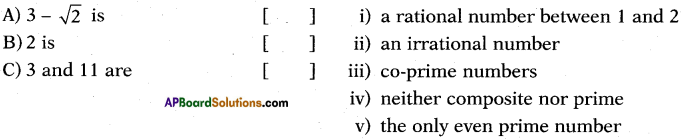A – (i), B – (iv)

Question 169.
Write the correct matching options :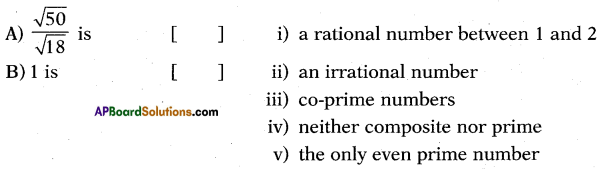A – (ii), B – (iii)

Question 170.
Write the correct matching options :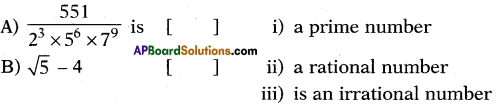A – (ii), B – (v)

Question 171.
Write the correct matching options: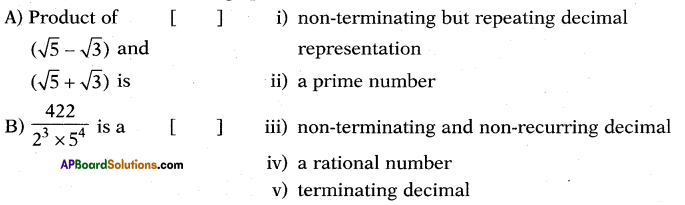A – (iii), B – (iv)

Question 172.
Write the correct matching options: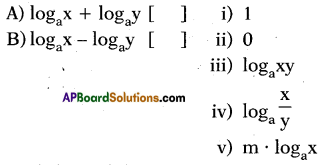A — (i), B – (ii), C — (v)

Question 173.
What is the value of $$\log _{\frac{2}{3}}\left(\frac{27}{8}\right)$$
-3

Question 174.
Write the decimal form of the rational number $$\frac{7}{2^{2} \times 5}$$
AP Model Paper
0.35Question 175.
What is the value of $$\log _{\sqrt{5}} \sqrt{5}$$ ?
Solution: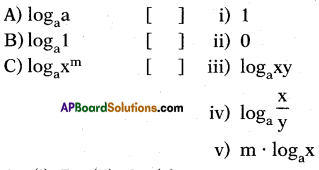Question 176.
Which statement do you agree with ? P: The product of two irrational num-bers is always a rational number.
Q : The product of a rational and an irrational number is always an irra-tional number,
i) Only P ii) Only Q iii) Both P and Q